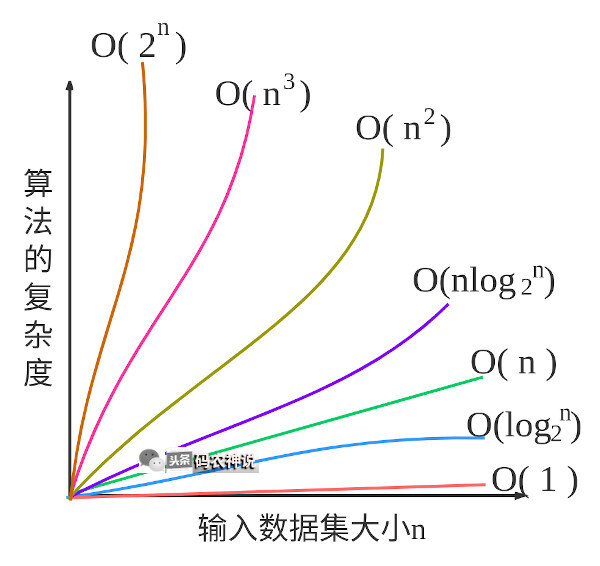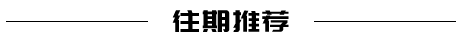# 什么是算法的大 O 表示法﻿

### 评价算法的指标

1. 与计算机的软硬件无关，硬件好理解，软件比如编程语言、执行器、编译器等等；

2. 每个语句所消耗的时间都一样，记作一个单位时间；

for (int i = 0; i < n; i++) { //①  for (int j = 0; j < n; j++) { //②    c[i][j] = 0; //③    for (int k = 0; k < n; k++) { //④      c[i][j] = a[i][k] + b[k][j] + c[i][j]; //⑤    }  }}

• 语句①循环到i=n时才会完结，所以它耗费n+1个时间单位；

• 语句②同语句①，自己的的循环中耗费n+1个时间单位，但它在①的循环n次中，所以消耗n*(n+1)个时间单位；

• 语句③在循环语句①②中，他们分别循环n次，所以语句③消耗n*n个时间单位；

• 语句④同③，但它本身执行n+1，所以语句④消耗n*n*(n+1)个时间单位；

• 语句⑤在循环语句①②④中，它消耗n*n*n个时间单位；

### 算法的时间复杂度

﻿

﻿

#### O(1)

O(1)表示算法总是执行相同的时间，比如

boolean isFirstElementEmpty(List<String> elements){  return elements.get(0).isEmpty();}

#### O(n)

O(n)表示算法是线性增长，其复杂度与数据集的大小成正比。

boolean ContainsValue(List<String> elements, String value) {  for (String element : elements) {    if (element.equals(value)) return true;  }  return false;}

boolean ContainsValue(List<String> elements, String value) {  int n = elements.size();  for (int i = 0; i < n; i++) {    if (elements.get(i).equals(value)) return true;  }  return false;}

#### O(n2)

O(n2)表示算法的复杂度与数据集大小的平方成正比，一般的循环嵌套就是这种，随着嵌套的层级可能是O(n3)、O(n4)等。

boolean ContainsDuplicates(List<String> elements) {  for (int i = 0; i < elements.size(); i++) {    for (int j = 0; j < elements.size(); j++) {      if (i == j) continue;      if (elements.get(i).equals(elements.get(j))) return true;    }  }  return false;}

#### O(2n)

O(2n)表示算法的复杂度与数据集大小成指数增长，比如递归

int Fibonacci(int number) {  if (number <= 1) return number;  return Fibonacci(number - 2) + Fibonacci(number - 1);}

#### O(log2n)指数复杂度

int binarySearch(int[] arr, int value) {  int start = 0, end = arr.length - 1;  while (start <= end) {    int middle = (start + end) / 2;    if (value == arr[middle]) {      return middle;    }    if (value > arr[middle]) {      start = middle + 1;    }    if (value < arr[middle]) {      end = middle - 1;    }  }  return -1;}

### 复杂度对比﻿

﻿﻿﻿## 评论